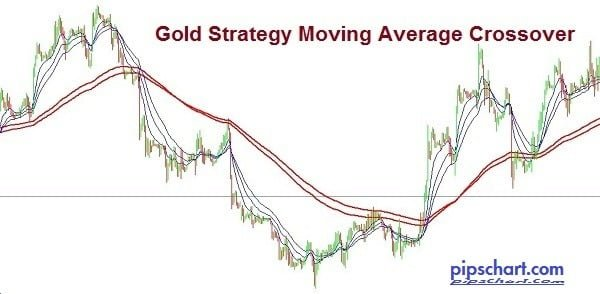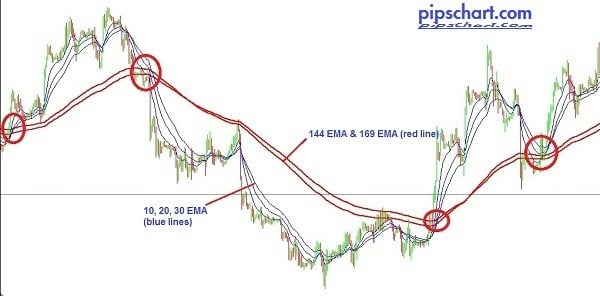# Gold Strategy : Moving Average Crossover

3,173 views

## Gold Strategy : Moving Average Crossover

The Gold Strategy Moving Average Crossover or Golden Cross is a trading technique that relies on the use of moving averages in the most volatile times of the trading session. It presents the best results during the sessions in London and New York.

Moving Average trading strategies is simplest form work based on the intersection of two moving averages, in which case an open position when the fastest average approaches and crosses the slower average as shown in the following example:There are multiple versions of the use of moving averages as a strategy to generate profits in the market. Using this technique we will show how to maximize profits and minimize risk by using these indicators.

The logic behind this technique is that during times of high volatility markets are at their best as they move with the greatest strength in accordance with the prevailing sentiment. During that time we will use a simple strategy with 5 moving average crosses special to show clear submit higher reliability.

### System Rules

• We use five exponential moving averages (EMA).
• EMA periods are: 10, 20, 30 and the values ​​of 144 and 169 Fibonacci.
• The first entry occurs when the EMA 10 crosses the two slower EMA (144 and 169 periods). In this case, the direction of the position (long or short) is the same as presented crossing moving averages.
• The second entry is the main trading. A position is opened after a price correction along with the intersection of 20 and 30 EMA on the slower periods EMA (144 and 169 periods).
• The target price depends on the type of chart.

### Example of Gold Strategy Moving Average Crossover• The blue lines are moving averages of 10, 20 and 30 periods.
• The red lines are moving averages of 144 and 169
• The red circles indicate the points where there was crossing the EMA 10 with the EMA 144 and 169 and then crossing the EMA 20 and EMA 30 with 144 and 169 first.

• 1st buy entry when the 144 and 169 EMA (red lines) crosses below the faster EMA 10 (red line) and price close above the cross.
• 2nd buy entry after a price correction along with the intersection of 20 and 30 EMA (red ines) on the slower periods EMA (144 and 169 periods).
• Stop loss set latest lower pick below the cross.
• Profit target should be 1:1 or 1:2.

Sell signal

• 1st sell entry when the 144 and 169 EMA (red lines) crosses above the faster EMA 10 (red line) and price close below the cross.
• 2nd sell entry after a price correction along with the intersection of 20 and 30 EMA (red ines) on the slower periods EMA (144 and 169 periods).
• Stop loss set latest higher pick above the cross.
• Profit target should be 1:1 or 1:2.

Here the example of Gold Strategy Moving Average Crossover buy and sell entry rules:Points to Remember

• Just be patient.
• But the crossing occurs not enter the market.
• The golden cross occurs only when the EMA 10, 20 and 30 cross the EMA 144 and 169 periods.
• Use levels Stop Loss and Take Profit according to the time frame in which it is trading. Follow the plan with discipline.

Tags

### 3 thoughts on “Gold Strategy : Moving Average Crossover”

1. Sudhan says:

In which timeframe it works?

2. Ernesto Garcia says:

Hi, very nice strategy! On what timeframes works best? Thanks!

• krT group says:

its depends on your region. 4H is the best for long time and 15M for short time.Thanks